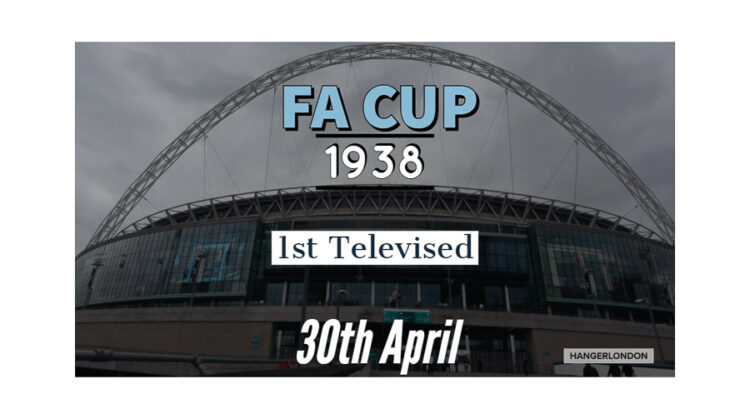# 30th April 1938 Football the 1st televised FA Cup

1938 The first televised FA Cup Huddersfield V Preston North End

London Picks on the Ebay Store....

## Maths Quiz: Test your basic Maths

/9
9
Created on

Mathematic Quiz - Prime and Composite Numbers

Math quiz helps us to increase our knowledge

1 / 9

is 441 PRIME number or a COMPOSITE number?

2 / 9

is 16 PRIME number or a COMPOSITE number?

3 / 9

is 908 PRIME number or a COMPOSITE number?

4 / 9

is 987 PRIME number or a COMPOSITE number?

5 / 9

is 29 PRIME number or a COMPOSITE number?

6 / 9

is 529 PRIME number or a COMPOSITE number?

7 / 9

is 601 PRIME number or a COMPOSITE number?

8 / 9

is 61 a Prime number or a Composite number?

9 / 9

is 850 PRIME number or a COMPOSITE number?

0%